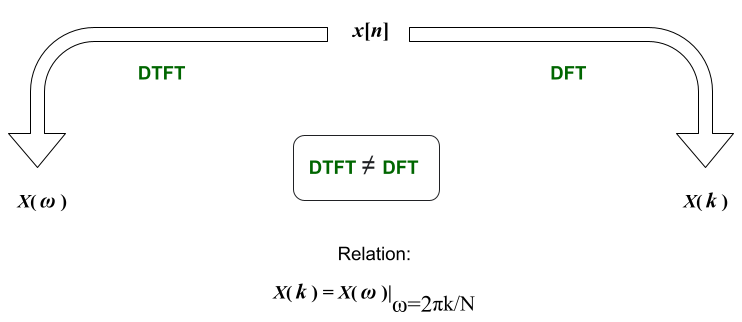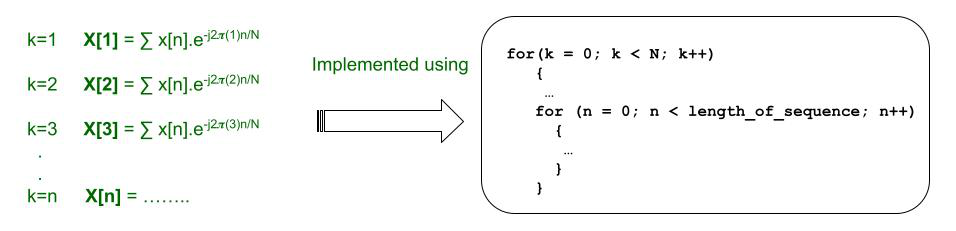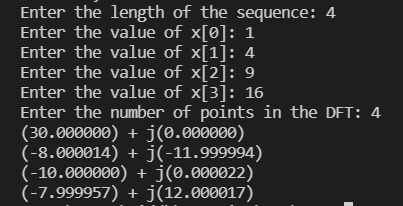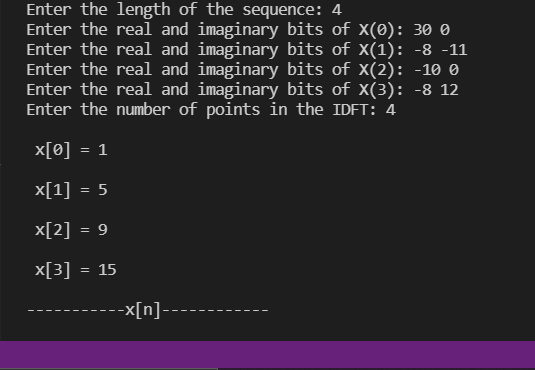# Discrete Fourier Transform and its Inverse using C

• Last Updated : 06 Jul, 2022

For decades there has been a provocation towards not being able to find the most perfect way of computing the Fourier Transform. Back in the 1800s, Gauss had already formulated his ideas and, a century later, so had some researchers, but the solution lay in having to settle with Discrete Fourier Transforms. It is a fairly good approximation by which one may get really close to depicting continuous-time signals and it works just fine (computers and the entire economy has been using it) even though it is finite and not time-limited or band-limited at the same time. What’s more, it doesn’t account for all the samples in the signal. Nevertheless, it works and is a fairly well-recognized success.

Discrete Fourier Transformation(DFT): Understanding Discrete Fourier Transforms is the essential objective here. The Inverse is merely a mathematical rearrangement of the other and is quite simple. Fourier Transforms is converting a function from the time domain to the frequency. One may assert that Discrete Fourier Transforms do the same, except for discretized signals. However, do not confuse this with Discrete-Time Fourier Transforms. The difference has been explained below:

• DFTs are calculated for sequences of finite length while DTFTs are for infinite lengths. This is why the summation in DTFTs ranges from -∞ to +∞.
• DTFTs are characterized by output frequencies that are continuous in nature, i.e., ω. DFTs, on the other hand, give an output that has discretized frequencies.
• DTFTs are equal to DFTs only for sampled values of ω. That is the only way by which we derive one from the other.The general expressions for DFT and IDFT are as follows. Note that the integral values of k are taken starting from 0 and counting till N-1. k is simply a variable used to refer to the sampled value of the function. However, since IDFT is the inverse of DFT, so k is not used. Instead, ‘n’ is used. Many find it confusing which is which. Take it as a stress-free activity to associate DFTs with a capital ‘X’ and IDFTs with the small case ‘x’.

Equation for DFT:Equation for IDFT:The first thing that comes to mind for coding the above expression is to start with a summation. In practice, this is achieved by running a loop and iterating over different values of n (in DFT) and k (in IDFT). Do take note of how one must also find different values of the output. When k=1, one may compute X[k=1] quite easily. However, in other applications, such as plotting the magnitude spectrum, one must compute the same for different values of k as well. Therefore, one must introduce two loops or a pair of nested loops.Yet another concern is how to translate the second half of the expression, which is the Euler’s constant raised to a complex exponent. Readers must recall the formula which helps describe the Euler’s constant raised to a complex number in terms of sines and cosines. This is as follows-This leaves us to interpret the second half of the summation term as follows-It is possible to import libraries (in the case of C) where one might have a problem with ensuring code legibility when it comes to writing this expression. However, a little mathematical insight and a simple conversion is the perfect requirement here. Many would agree. Note that this gives rise to imaginary and real parts of the expression – cosine terms are real and sine terms are all imaginary.  A rather intuitive perspective may be implemented as well – express the sequences as matrices and use the vector form of DFT and IDFT for calculations. This is best worked out in MATLAB.

Algorithm (DFT):

• Initialize all required libraries.
• Prompt the user to input the number of points in the DFT.
• Now you may initialize the arrays and accordingly ask for the input sequence. This is purely due to the inability to declare an empty array in C. Dynamic memory allocation is one of the solutions. However, simply reordering the prompt is a fair solution in itself.
• Implement 2 loops that calculate the value of X(k) for a specific value of k and n. Keep in mind that Euler’s formula will be used to substitute for e-j2kπn/N. This requires a division where we calculate the real and imaginary bits of the expression separately.
• Display the result as you run the calculation.

Below is the C program to implement the above approach:

## C

 // C program for the above approach#include #include  // Function to calculate the DFTvoid calculateDFT(int len){    int xn[len];    float Xr[len];    float Xi[len];    int i, k, n, N = 0;     for (i = 0; i < len; i++) {         printf("Enter the value "               "of x[%d]: ",               i);        scanf("%d", &xn[i]);    }     printf("Enter the number of "           "points in the DFT: ");    scanf("%d", &N);    for (k = 0; k < N; k++) {        Xr[k] = 0;        Xi[k] = 0;        for (n = 0; n < len; n++) {            Xr[k]                = (Xr[k]                   + xn[n] * cos(2 * 3.141592 * k * n / N));            Xi[k]                = (Xi[k]                   - xn[n] * sin(2 * 3.141592 * k * n / N));        }         printf("(%f) + j(%f)\n", Xr[k], Xi[k]);    }} // Driver Codeint main(){    int len = 0;    printf("Enter the length of "           "the sequence: ");    scanf("%d4", &len);    calculateDFT(len);     return 0;}

Input:

>> Enter the length of the sequence: 4
>> Enter the value of x: 1
>> Enter the value of x: 4
>> Enter the value of x: 9
>> Enter the value of x: 16
>> Enter the number of points in the DFT: 4

Output:Algorithm (IDFT):

• Initialize all required libraries.
• Prompt the user to enter the length of the sequence. This shall be substituted as the value of N. Initialize the arrays responsible for storing the real and imaginary parts of the input.
• Now obtain the real and imaginary parts of the sequence one by one using a ‘for’ loop. Remember that we are literally reversing the process defined for DFT calculations.
• Define theta. Theta is the exponent to which the e is raised in Euler’s conversion of e i.e., theta = 2kπn/N.
• Calculate x[n] using cosines and sines. Take care while using the signs involved in the expressions.
• Divide the obtained output by the length or multiply by 1/N and print the result.

Below is the C program to implement the above approach:

## C

 // C program for the above approach #include #include  // Function to calculate the inverse// discrete fourier transformationvoid calculate_IDFT(int len){    int x[len];    float Xr[len];    float Xi[len];    int i, k, n, N = 0;    for (i = 0; i < len; i++) {        printf(            "Enter the real and "            "imaginary bits of X(%d): ",            i);        scanf("%f %f", &Xr[i], &Xi[i]);    }     printf("Enter the number of "           "points in the IDFT: ");    scanf("%d", &N);     for (n = 0; n < N; n++) {        x[n] = 0;        for (k = 0; k < N; k++) {            int theta = (2 * 3.141592 * k * n) / N;            x[n] = x[n] + Xr[k] * cos(theta)                   + Xi[k] * sin(theta);        }        x[n] = x[n] / N;        printf("\n x[%d] = %d\n", n,               x[n]);    }     printf("\n-----------x[n]------------\n\n");} // Driver Codeint main(){    int len = 0;    printf("Enter the length of "           "the sequence: ");    scanf("%d", &len);    calculate_IDFT(len);     return 0;}

Input:

>> Enter the length of the sequence: 4
>> Enter the real and imaginary bits of X(0): 30 0
>> Enter the real and imaginary bits of X(1): -8 -11
>> Enter the real and imaginary bits of X(2): -10 0
>> Enter the real and imaginary bits of X(3): -8 12
>> Enter the number of points in the IDFT: 4

The output of the DFT obtained earlier (not exactly; expect some deviation) is used as the input for the IDFT.

Output:My Personal Notes arrow_drop_up